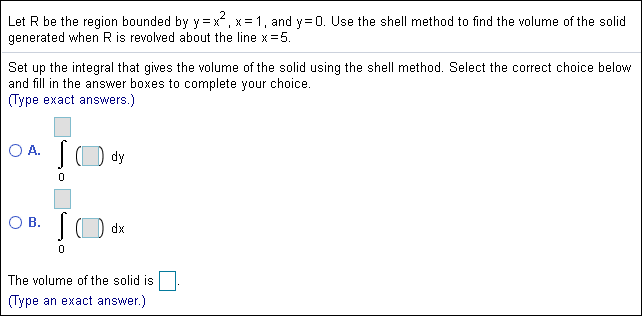# 2Let R be the region bounded by Y = x x 1, and y 0. Use the shell method to find the volume of the solidgenerated when R is revolved about the line x 5.Set up the integral that gives the volume of the solid using the shell method. Select the correct choice belowand fill in the answer boxes to complete your choice.Type exact answers.)O A.dy0O B.dxThe volume of the solid isType an exact answer.)

Question
94 views

:)help_outlineImage Transcriptionclose2 Let R be the region bounded by Y = x x 1, and y 0. Use the shell method to find the volume of the solid generated when R is revolved about the line x 5. Set up the integral that gives the volume of the solid using the shell method. Select the correct choice below and fill in the answer boxes to complete your choice. Type exact answers.) O A. dy 0 O B. dx The volume of the solid is Type an exact answer.) fullscreen
check_circle

Step 1

Given:

The given curves are y = x2, x = 1, and y = 0.

The volume of the solid revolved around the line x = 5. So, the radius of the cylinder is 5 – x.

The height of the cylinder is the difference of the functions y = x2 and y = 0. That is, h = x2.

Step 2

Calculation:

Use shell method to find the volume of the solid generated when R is revolved about the line x = 5 is calculated as follows.

Step 3

Calculate the cross-section...

### Want to see the full answer?

See Solution

#### Want to see this answer and more?

Solutions are written by subject experts who are available 24/7. Questions are typically answered within 1 hour.*

See Solution
*Response times may vary by subject and question.
Tagged in

### Other